# Moment of inertia of continuous bodies - uniform rod along with the axis passing through the center of mass

Moment of inertia of continuous bodies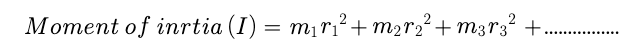Lets take an exmaple:

If the mass is continuous distributed over the body as show in below figure :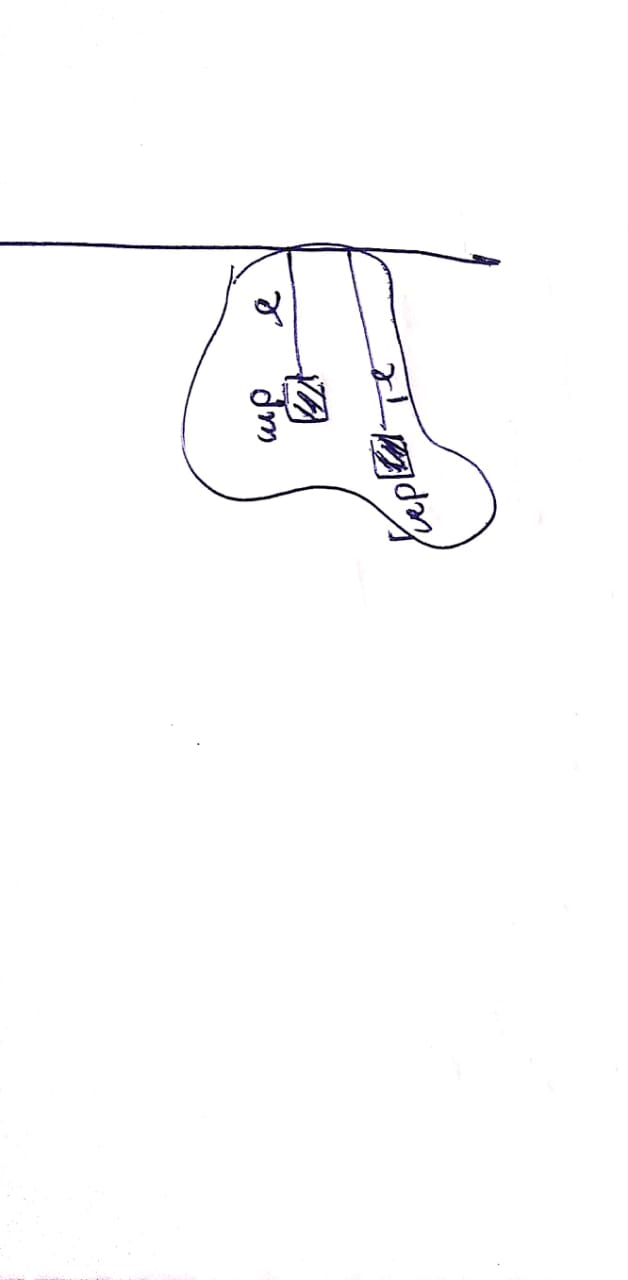lets a mass of small portion at distance of r from the axis. so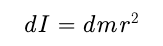now for calculate the moment of interia of whole body, we have to sum of all small portion of moment of interia with the help of integration so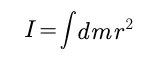here we have to find the range of  r to caculte the moment of inertia of whole body so the formula isLet take an another example :

Calculte the moment of inertia of uniform rod along with the axis passing through the center of mass as shown in the figure.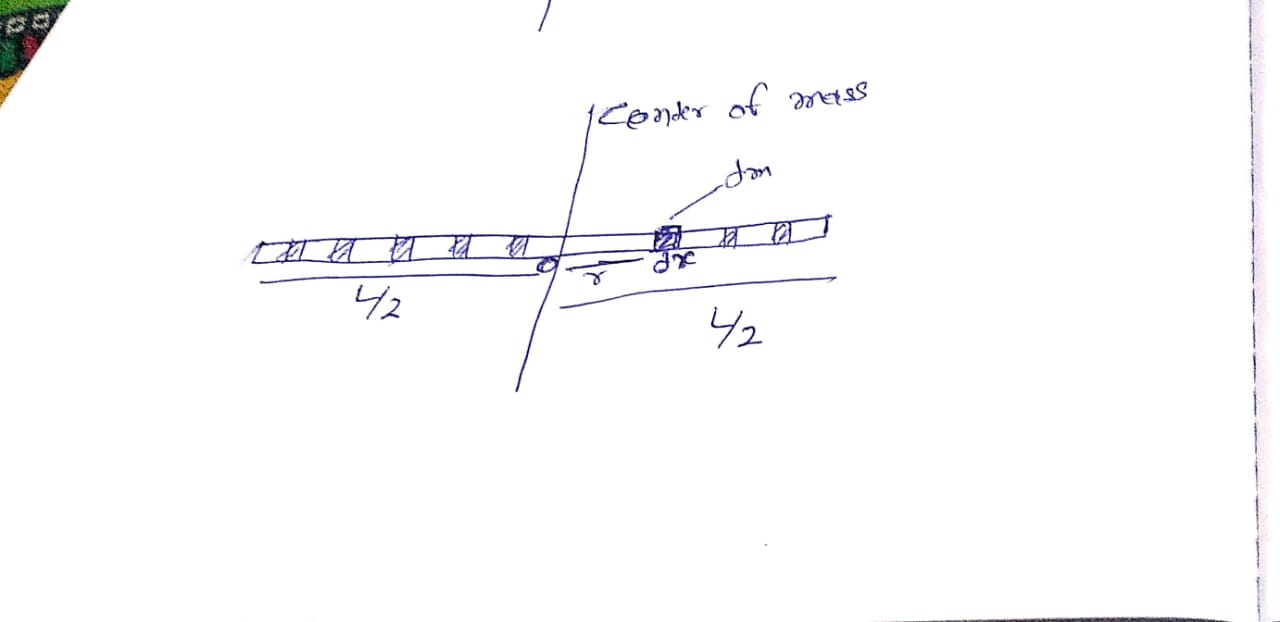Lets take mass of rod is M and length is L and it is one dimention rod thats which we can neglect the thickness of the rod.now calculte the range of r as shown in figure r is range from -r/2 to +r/2now the question is how to calculate dm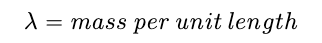mass of 1m length is  =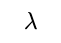mass of 2m length is  =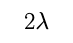mass of dx m length =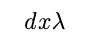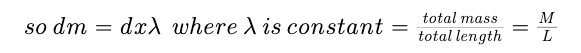07 Jun, 2019 2614 views Physics